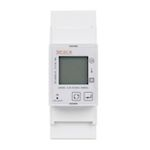# TuYa TS0601_3_phase_clamp_meter_relay

Model TS0601_3_phase_clamp_meter_relay
Vendor TuYa
Description 3-phase clamp power meter with relay
Exposes switch (state), power, energy, produced_energy, voltage_a, voltage_b, voltage_c, power_a, power_b, power_c, current_a, current_b, current_c, linkquality
PictureWhite-label Wenzhou Taiye Electric TAC7361C BI

## Options

How to use device type specific configuration

• `energy_precision`: Number of digits after decimal point for energy, takes into effect on next report of device. The value must be a number with a minimum value of `0` and with a with a maximum value of `3`

• `energy_calibration`: Calibrates the energy value (percentual offset), takes into effect on next report of device. The value must be a number.

• `power_precision`: Number of digits after decimal point for power, takes into effect on next report of device. The value must be a number with a minimum value of `0` and with a with a maximum value of `3`

• `power_calibration`: Calibrates the power value (percentual offset), takes into effect on next report of device. The value must be a number.

## Exposes

### Switch

The current state of this switch is in the published state under the `state` property (value is `ON` or `OFF`). To control this switch publish a message to topic `zigbee2mqtt/FRIENDLY_NAME/set` with payload `{"state": "ON"}`, `{"state": "OFF"}` or `{"state": "TOGGLE"}`. It’s not possible to read (`/get`) this value.

### Power (numeric)

Instantaneous measured power. Value can be found in the published state on the `power` property. It’s not possible to read (`/get`) or write (`/set`) this value. The unit of this value is `W`.

### Energy (numeric)

Sum of consumed energy. Value can be found in the published state on the `energy` property. It’s not possible to read (`/get`) or write (`/set`) this value. The unit of this value is `kWh`.

### Produced_energy (numeric)

Sum of produced energy. Value can be found in the published state on the `produced_energy` property. It’s not possible to read (`/get`) or write (`/set`) this value. The unit of this value is `kWh`.

### Voltage_a (numeric)

Measured electrical potential value (phase A). Value can be found in the published state on the `voltage_a` property. It’s not possible to read (`/get`) or write (`/set`) this value. The unit of this value is `V`.

### Voltage_b (numeric)

Measured electrical potential value (phase B). Value can be found in the published state on the `voltage_b` property. It’s not possible to read (`/get`) or write (`/set`) this value. The unit of this value is `V`.

### Voltage_c (numeric)

Measured electrical potential value (phase C). Value can be found in the published state on the `voltage_c` property. It’s not possible to read (`/get`) or write (`/set`) this value. The unit of this value is `V`.

### Power_a (numeric)

Instantaneous measured power (phase A). Value can be found in the published state on the `power_a` property. It’s not possible to read (`/get`) or write (`/set`) this value. The unit of this value is `W`.

### Power_b (numeric)

Instantaneous measured power (phase B). Value can be found in the published state on the `power_b` property. It’s not possible to read (`/get`) or write (`/set`) this value. The unit of this value is `W`.

### Power_c (numeric)

Instantaneous measured power (phase C). Value can be found in the published state on the `power_c` property. It’s not possible to read (`/get`) or write (`/set`) this value. The unit of this value is `W`.

### Current_a (numeric)

Instantaneous measured electrical current (phase A). Value can be found in the published state on the `current_a` property. It’s not possible to read (`/get`) or write (`/set`) this value. The unit of this value is `A`.

### Current_b (numeric)

Instantaneous measured electrical current (phase B). Value can be found in the published state on the `current_b` property. It’s not possible to read (`/get`) or write (`/set`) this value. The unit of this value is `A`.

### Current_c (numeric)

Instantaneous measured electrical current (phase C). Value can be found in the published state on the `current_c` property. It’s not possible to read (`/get`) or write (`/set`) this value. The unit of this value is `A`.

Link quality (signal strength). Value can be found in the published state on the `linkquality` property. It’s not possible to read (`/get`) or write (`/set`) this value. The minimal value is `0` and the maximum value is `255`. The unit of this value is `lqi`.